# Pointers

Whenever you reference the name of a variable, the name of an element of a structure, or the name of an attribute of a class, you are telling the computer that you want to do something with the contents stored at the corresponding memory location.

In the first statement in the following example, the computer is told to store the letter A into the memory location represented by the variable grade . The last statement tells the computer to copy the contents of the memory location represented by the grade variable and store it in the memory location represented by the oldGrade variable.

` char grade = 'A'; char oldGrade; oldGrade = grade; `

A pointer is a variable and can be used as an element of a structure and as an attribute of a class in some programming languages such as C++, but not Java. However, the contents of a pointer is a memory address of another location of memory, which is usually the memory address of another variable, element of a structure, or attribute of a class.

### Declaring a Pointer

A pointer is declared similar to how you declare a variable but with a slight twist. The following example declares a pointer called ptGrade . There are four parts of this declaration:

• Data type The data type of the memory address stored in the pointer

• Asterisk (*) Tells the computer that you are declaring a pointer

• Variable name The name that uniquely identifies the pointer and is used to reference the pointer within your program

• Semicolon Tells the computer this is an instruction (statement)

` char *ptGrade; `

### Data Type and Pointers

As you will recall, a data type tells the computer the amount of memory to reserve and the kind of data that will be stored at that memory location. However, the data type of a pointer tells the computer something different. It tells the computer the data type of the value at the memory location whose address is contained in the pointer.

Confused? Many programmers are confused about the meaning of the data type used to declare a pointer, so you re in good company. The best way to clear any confusion is to get back to basics.

The asterisk (*) used when declaring a pointer tells the computer the amount of memory to reserve and the kind of data that will be stored at that location. That is, the memory size is sufficient to hold a memory address, and the kind of data stored there is a memory address.

You re probably wondering why you use a data type when declaring a pointer. Before answering that question, let s make sure you have a firm understanding of pointers. The following example declares four variables . The first statement declares an integer variable called studentNumber . The second statement declares a char variable called grade . The last two statements each declare a pointer. Figure 2-3 depicts memory reserved by these statements. Assume that a memory address is 4 bytes for this example.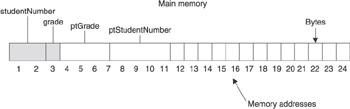Figure 2-3: Memory allocated when two pointers and two variables are declared
` int studentNumber; char grade; char *ptGrade; int *ptStudentNumber; `

The char data type in the ptGrade pointer declaration tells the computer that the address that will be stored in ptGrade is the address of a character. As you ll see in the next section, the contents of the memory location associated with ptGrade will contain the address of the grade variable.

Likewise, the int data type of the ptStudentNumber pointer states that the contents of the memory location associated with ptStudentNumber will contain the address of an integer variable, which will be the address of the studentNumber variable.

Why does the computer need to know this? For now, let s simply say that programmers instruct the computer to manipulate memory addresses using pointer arithmetic. In order for the computer to carry out those instructions, the computer must know the data type of the address contained in a pointer. You ll learn pointer arithmetic later in this chapter.

### Assigning an Address to a Pointer

An address of a variable is assigned to a pointer variable by using the address operator (&). Before you learn about dereferencing a variable, let s review an assignment statement. The following assignment statement tells the computer to copy the value stored at the memory location that is associated with the grade variable and store the value into the memory location associated with the oldGrade variable:

` oldGrade = grade; `

An assignment statement implies that you want the contents of a variable and not the address of the variable. The address operator tells the computer to ignore the implied assignment and assign the memory address of the variable and not the content of the variable.

The next example tells the computer by using the address operator to copy the address of the variable to the pointer variable. That is, the memory address of the grade variable is copied to the ptGrade pointer variable, and the memory address of the studentNumber variable is assigned to the ptStudentNumber pointer:

` ptGrade = &grade; ptStudentNumber = &studentNumber; `

Figure 2-4 depicts memory after the previous two statements execute. Notice that the grade variable is an alias for memory address 3 and the studentNumber variable is the alias for memory address 1. The content of ptGrade pointer is 3, which is the memory address of the grade variable. Likewise, the content of pointer ptStudentNumber is 1, which is the memory address of studentNumber .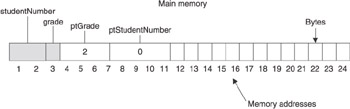Figure 2-4: Memory allocated after pointers are assigned memory addresses

### Accessing Data Pointed to by a Pointer

A pointer variable references a memory location that contains a memory address. Sometimes a programmer wants to copy that memory address to another pointer variable. This is accomplished by using an assignment statement as shown here:

` ptOldGrade = ptNewGrade; `

You ll notice that this assignment statement is identical to assignment statements used with any variable. Remember that the assignment statement tells the computer to copy the contents of a variable regardless if the content is a memory address or any other value.

Other times, programmers want to the use the content of the memory address stored in the pointer variable. This may be tricky to understand, so let s look at an example to clear up any confusion. The following statements will be familiar to you because we ve used them in examples throughout this chapter.

The first two statements declare variables, one of which is initialized with a value. The next two statements declare pointer variables. And the last statement assigns the address of the first variable to pointer variables. Figure 2-5 shows how memory looks after these statements execute.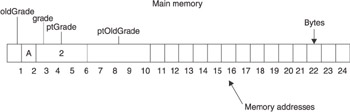Figure 2-5: Memory allocated after values are assigned to variables
` char oldGrade; char grade = 'A'; char *ptGrade; char *ptOldGrade; ptGrade = &grade; `

Let s say a programmer wants to use the value stored in the grade variable to display the grade on the screen. However, the programmer wants to use only the ptGrade pointer to do this. Here s how it is done.

The programmer uses the pointer dereferencing operator (sometimes called the dereferencing operator), which is the asterisk (*), to dereference the point variable. Think of dereferencing as telling the computer you are referring to to go to the memory address contained in the pointer and then perform the operation. Without dereferencing, the computer is told to use the contents of the pointer when performing the operation.

Let s say that you want to copy the content of ptGrade to ptOldGrade . Here s how you would do it:

` ptOldGrade = ptGrade; `

Figure 2-6 shows you the effect this statement has on memory.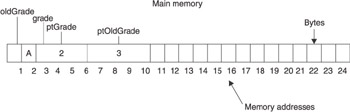Figure 2-6: Memory allocated after the value of the ptGrade is copied to ptOldGrade.

Now let s suppose you want to copy the contents of grade to the oldGrade variable, but you only want to use the ptGrade pointer. You do this by dereferencing the ptGrade pointer variable using the asterisk (*) as the dereferencing pointer operator as shown here:

` char oldGrade = *ptGrade; `

The previous statement tells the computer to go to the memory address contained in the ptGrade pointer variable and then perform the assignment operation, which copies the value of memory address 2 to the memory address represented by the oldGrade variable, which is memory address 1. The result is shown in Figure 2-7.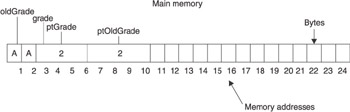Figure 2-7: Memory allocated after the contents of the memory address pointed to by ptGrade is copied to the oldGrade variable.

You can dereference a pointer variable any time you want to use the contents of the memory address pointed to by the variable and use the dereference pointer variable in any statement that you would use a variable.

### Pointer Arithmetic

Pointers are used to step through memory sequentially by using pointer arithmetic and the incremental (++) or decremental ( - - ) operator. The incremental operator increases the value of a variable by 1, and the decremental operator decreases the value of a variable by 1.

In the following example, the value of the studentNumber variable is incremented by 1, making the final value 1235.

` int studentNumber = 1234; studentNumber++; `

Likewise, the next example decreases the value of the studentNumber variable by 1, resulting in the final value of 1233.

` int studentNumber = 1234; studentNumber--; `

Pointer arithmetic uses the incremental and decremental operator in  a similar but slightly different way. The following statements declare two variables used to store student numbers and two pointers each pointing to one of those variables. Figure 2-8 depicts memory allocation after these statements execute.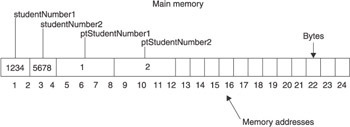Figure 2-8: Memory allocation before incrementing ptStudentNumber1
` int studentNumber1 = 1234; int studentNumber2 = 5678; int *ptStudentNumber1; int *ptStudentNumber2; ptStudentNumber1 = &studentNumber1; ptStudentNumber2 = &studentNumber2; `

What would be the value stored in the pointer variable studentNumber1 if the studentNumber1 is incremented by 1 using the following statement?

` ptStudentNumber1++; `

This is tricky because the value of ptStudentNumber1 is 0. If you increment it by one, the new value is 1. However, memory address 2 is the second half of the memory location reserved for studentNumber1 . This means that ptStudentNumber1 would point to the middle of the values of studentNumber1 , which doesn t make sense.

That s not what happens. The computer uses pointer arithmetic. Values are incremented and decremented in pointer arithmetic using the size of a data type. That is, if the memory address contains an integer and the memory address is incremented, the computer adds the size of an integer to the current memory address.

Let s return to Figure 2-8 and see how this works. ptStudentNumber1 contains the memory address 1. If you go to memory address 1, you ll notice that the memory address stores an integer. In the example, the size of an integer is 2 bytes. When ptStudentNumber1 is incremented using pointer arithmetic, the computer adds 2 bytes to the address stored in ptStudentNumber1 making the new value 2, which is stored in ptStudentNumber1 . Figure 2-9 shows the results of incrementing using pointer arithmetic.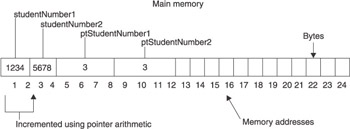Figure 2-9: Memory allocation after incrementing ptStudentNumber1

Decrementing a value using pointer arithmetic is very similar to incrementing a value, except the size of a data type is subtracted from the value. Let s return to Figure 2-8 for a moment. If the following statement executed, the value of ptStudentNumber2 would be 1 because the computer subtracts the size of an integer (2 bytes) from the current value of the ptStudentNumber2 (2).

` ptStudentNumber2--; `

### Pointers to Pointers

Imagine having a list of a million students along with their final grades and student numbers and being asked to sort the list by last name, first name, and student number. Intuitively, you might think about making two copies of this list, each placed in one of the sort orders. However, this wastes memory. There is a better approach to sort the list:  use pointers to pointers.

You learned that a pointer is a variable that contains the memory address of another variable. A pointer to a pointer is also a variable that contains the memory address, except a pointer to a pointer contains the memory address of another pointer variable.

Confused? You re not alone. The concept of a pointer to a pointer isn t intuitive to understand. However, we can clear up any confusion by declaring variables and storing values into memory.

Let s begin by declaring four char variables and initializing them with letters of the alphabet. This is shown in the first statement of the following example. The second statement declares a pointer called ptInitial and a pointer to a pointer called ptPtInitial . A pointer is declared using a signal asterisk (*). A pointer to a pointer is declared using a double asterisk (**).

` char inital1 = 'D', inital2 = 'A', inital3 = 'C', inital4 = 'B'; char *ptInitial, **ptPtInitial; ptInitial = &inital1; ptPtInitial = &ptInitial; `

With variables declared, the next two statements assign values to the pointer and to the pointer to a pointer. In both cases, the ampersand (&) is used as the dereferencing operator.

The ptInitial pointer variable is assigned the address of variable inital1 , which is memory address 1. The ptPtInitial pointer to a pointer variable is assigned the memory address of ptInitial . The address of ptInitial is memory address 5. Figure 2-10 shows the allocated memory after these statements execute.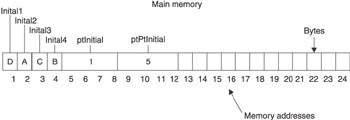Figure 2-10: The pointer to a pointer variable is assigned the memory address of the ptInitial pointer.

Programmers use a pointer to a pointer to tell a computer to use the contents of the memory address contained in the pointer variable that the pointer to a pointer is pointing to. This is a mouthful, so we ll restate this using an example:

You can use the content of the inital1 variable by referencing the ptPtInitial variable. Here s how this is done:

` cout << **ptPtInitial; `

The cout statement is used in C++ to display something on the screen. In this example, you re displaying the content of the initial1 variable, although it doesn t seem to be doing so. This statement is telling the computer to go to the memory address stored in the ptPtInitial pointer to a pointer variable, which is memory address 5 (see Figure 2-11). The content of that memory address is another memory address, which is memory address 1. The computer is told to go to memory address 1 and display the content of that memory address, which is the letter D .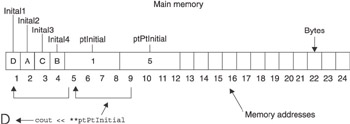Figure 2-11: Two memory addresses are referenced when using a pointer to a pointer to display a value on the screen.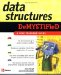Data Structures Demystified (Demystified)
ISBN: 0072253592
EAN: 2147483647
Year: 2006
Pages: 90

Similar book on Amazon